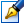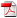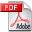##Polling models with priorities, semi-Markov vacations and exhaustive service

 Author: Mitev Lilia Degree: doctor of Speciality: 01.01.09 - Mathematical cybernetics and operation research Year: 2017 Scientific adviser: Gheorghe Mişcoidoctor habilitat, professor, Institute of Mathematics and Computer Science Institution: Institute of Mathematics and Computer Science

### Status

The thesis was presented on the 23 March, 2017
Approved by NCAA on the 31 May, 2017

### Abstract0.86 Mb / in romanian

### Thesis

CZU 519.8722.70 Mb / in romanian
145 pages

### Keywords

Polling model, generalized queueing models with priorities, Laplace-Stieltjes transform, virtual queue length, busy period, DD priority

### Summary

This thesis is submitted to obtain a doctoral degree in mathematics, at the specialty 112.03 - Mathematical cybernetics and operational research. It was elaborated at University of the Academy of Sciences of Moldova and Institute of Mathematics and Computer Science of the Academy of Sciences of Moldova, in Chisinau, 2016

. Thesis structure. The thesis is written in Romanian and contains the following sections: introduction, three chapters, conclusions, bibliography consisting of 103 titles and two annexes. The text of thesis comprises 108 pages. The results of thesis are published in 30 scientific papers.

The field of study of the thesis: Queueing theory. The aim of research. The paper aims to extend the known results from Queueing theory, the development of new techniques and numerical algorithms for determining optimal performance characteristics for Polling models with semi-Markov vacations and for those with DD priority. To achieve the intended goal has been achieved the following objectives:
• elaboration and application of numerical algorithms for modeling the distribution of virtual queue length of the Polling systems with semi-Markov vacations;
• formalization of probabilistic performance characteristics for generalized queueing systems with DD priority;
• development and application of numerical algorithms for modeling the distribution of busy period and of auxiliary characteristics for the Polling systems with DD priority;
• implementation of developed algorithms in programming languages in order to estimate parameters of distribution functions that occur in optimization of performance characteristics of the Polling queueing models.

Scientific novelty and originality: consists in the elaboration of methods and numerical algorithms for determination of numerical performance characteristics for Polling systems, and for those with DD priority. Thus it can determine the efficiency / performance of the queueing system depending on distribution laws, priority laws, strategy of the system in the free state.

The important scientific solved problem: resides in the determination of more optimal values of probabilistic characteristics for Polling models, the obtained new results both from analysis queueing models and distribution functions, priority laws, schemes of service and orientation, which allows the establishment stationarity and efficiency of the queueing system

.

The theoretical significance. The obtained results from the thesis can be used to study some real systems where queueing phenomena occur and assessment of other numeric characteristics. Applicative value of the thesis.

The presented results permit application in various spheres, where queueing phenomena occur, similar to studied, such as computer systems, telecommunications, economic, etc., which can be modeled using studied mathematical models. The implementation of the scientific results. The developed algorithms were implemented in programming languages C++ and Kotlin.